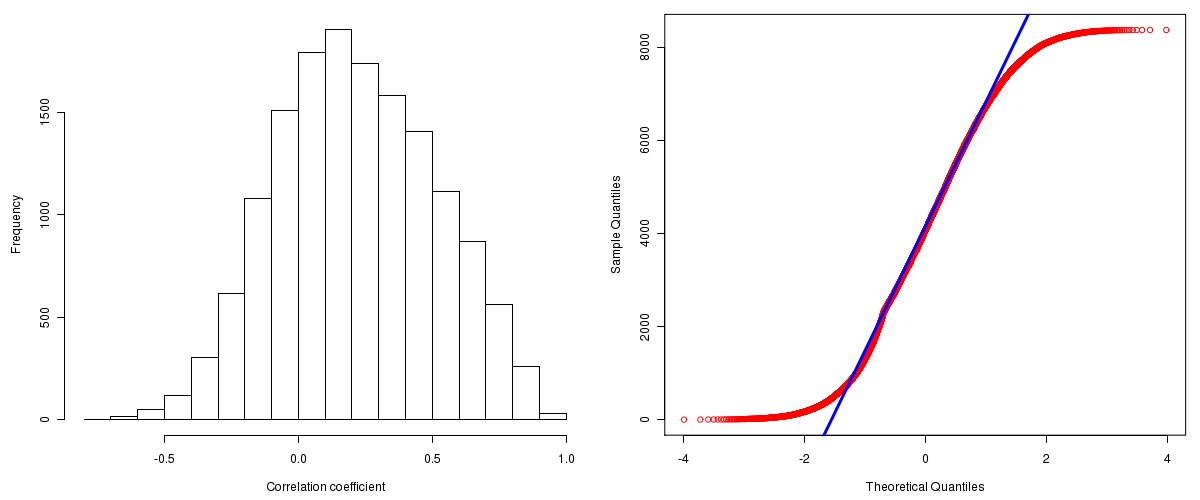Brain Lower Grade Glioma: Correlations between copy number and mRNA expression
Maintained by John Zhang (Dana-Farber Cancer Institute)
Overview
Introduction

A TCGA sample is profiled to detect the copy number variations and expressions of genes. This pipeline attempts to correlate copy number and expression data of genes across samples to determine if the copy number variations also result in differential expressions. This report contains the calculated correlation coefficients based on measurements of genomic copy number (log2) values and intensity of the expressions of the corresponding feature across patients. High positive/low negative correlation coefficients indicate that genomic alterations result in differences in the expressions of mRNA the genomic regions transcribe.

Summary

The correlation coefficients in 10, 20, 30, 40, 50, 60, 70, 80, 90 percentiles are -0.15638, -0.0426, 0.0455, 0.1246, 0.2043, 0.2886, 0.3832, 0.48898, 0.62524, respectively.

Results
Correlation results

Number of genes and samples used for the calculation are shown in Table 1. Figure 1 shows the distribution of calculated correlation coefficients and quantile-quantile plot of the calculated correlation coefficients against a normal distribution. Table 2 shows the top 20 features ordered by the value of correlation coefficients.

Table 1.  Counts of mRNA and number of samples in copy number and expression data sets and common to both

Category Copy number Expression Common
Sample 80 27 27
Genes 29390 17815 15551

Figure 1.  Summary figures. Left: histogram showing the distribution of the calculated correlations across samples for all Genes. Right: QQ plot of the calculated correlations across samples. The QQ plot is used to plot the quantiles of the calculated correlation coefficients against that derived from a normal distribution. Points deviating from the blue line indicate deviation from normality.Table 2.  Get Full Table Top 20 features (defined by the feature column) ranked by correlation coefficients

feature r p-value q-value chrom start end geneid
MRPS15 0.9523 2.1316282072803e-14 1.74993660206606e-10 1 36693960 36702556 64960
CSDE1 0.9473 7.32747196252603e-14 3.00770353480104e-10 1 115061060 115102147 7812
C1orf142 0.9451 1.20348175869367e-13 3.29328346638821e-10 1 225989320 226035550 116841
APITD1 0.9402 3.39062111720523e-13 6.95873226915333e-10 1 10412746 10434797 378708
LSM10 0.9343 1.06581410364015e-12 1.46223001141388e-09 1 36631618 36636080 84967
POP4 0.9343 1.06870068350418e-12 1.46223001141388e-09 19 34789041 34798547 10775
PPP1R15B 0.9276 3.45368178500394e-12 4.05037409353207e-09 1 202639143 202647542 84919
GPBP1L1 0.9248 5.46118705813115e-12 5.60412639685089e-09 1 45865567 45899398 60313
TRIM8 0.9201 1.14126486039368e-11 1.04100973927073e-08 10 104394356 104408065 81603
C19orf12 0.9192 1.2958079054215e-11 1.06377916899345e-08 19 34883910 34897971 83636
RRAGA 0.9185 1.44737555274332e-11 1.08018813891169e-08 9 19039372 19041023 10670
NRD1 0.9158 2.12532214050043e-11 1.28688393341936e-08 1 52027470 52117010 4898
PARP11 0.9157 2.1674662065152e-11 1.28688393341936e-08 12 3788288 3852863 57097
PSMD8 0.9154 2.27566854249517e-11 1.28688393341936e-08 19 43557061 43566304 5714
NOL1 0.9151 2.35136354831411e-11 1.28688393341936e-08 12 6536298 6547741 4839
PSMC1 0.9116 3.85949050496492e-11 1.9614301654187e-08 14 89792647 89808719 5700
C12orf4 0.9112 4.06172873113064e-11 1.9614301654187e-08 12 4467162 4517898 57102
PEX14 0.909 5.45519185379817e-11 2.48798856710411e-08 1 10457606 10613400 5195
FLJ10241 0.9072 6.85473899864064e-11 2.96174851109547e-08 19 46629064 46637650 55101
DNAJC16 0.9047 9.44253564227893e-11 3.69130724094743e-08 1 15725895 15767447 23341
Methods & Data
Input

Gene level (TCGA Level III) expression data and copy number data of the corresponding loci derived by using the CNTools package of Bioconductor were used for the calculations. Pearson correlation coefficients were calculated for each pair of genes shared by the two data sets across all the samples that were common.

Correlation across sample

Pairwise correlations between the log2 copy numbers and expressions of each gene across samples were calculated using Pearson correlation.